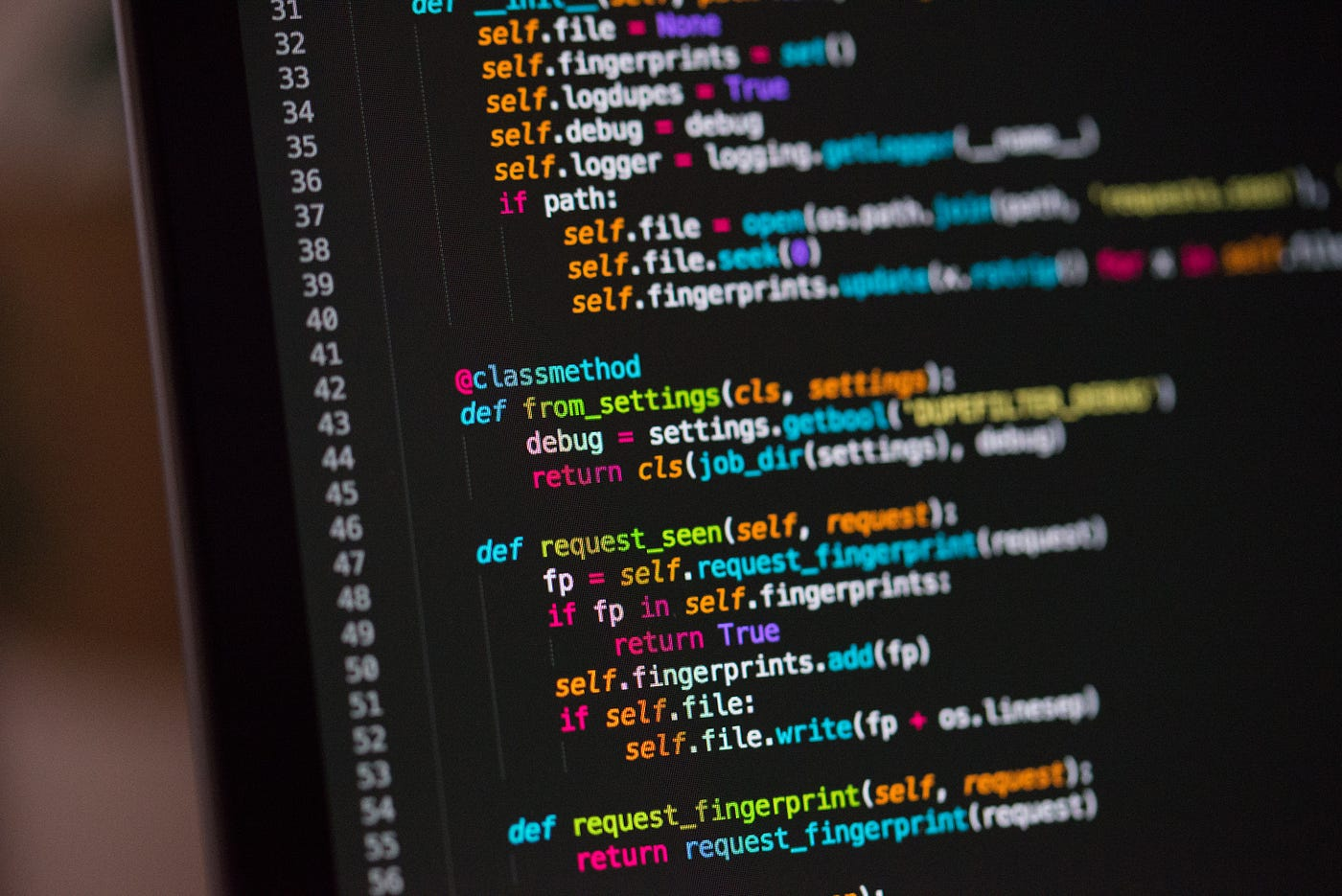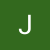# The Blind 75 Leetcode Series: Maximum SubarrayPhoto by Chris Ried on Unsplash

Today, we will be working on 53. Maximum Subarray.

Given an integer array `nums`, find the contiguous subarray (containing at least one number) which has the largest sum and return its sum.

A subarray is a contiguous part of an array.

We have a list of numbers, can be positive, zero, or negative. We want to find the sub-list that maximizes the sum. Some questions to ask would be

1. do we deal with empty list? If so, what do we return?
2. Is the list finite? We want to know this to see if we need to keep tracking the max.

Since the question also comes with constraints

`1 <= nums.length <= 105`

`-104 <= nums[i] <= 104`

We can say that the list is always present, and it is finite.

First approach is again the brute force. We check every possible subarray and find the max. Since we don’t need to record the array but only the max, a simple integer is good enough for this.

`def maxSubArray(nums):    max_sum = float('-inf')  # initialize as -inf so any sum is greater    for i in range(len(nums)):        for j in range(i, len(nums)):            max_sum = max(max_sum, sum(nums[i:j+1]))  # inclusive on the right    return max_sum`

Simple, right? What’s the time complexity for this? We have 2 for-loops nested, so if you’ve been following this series and/or have been working on problems, you can see that it’s O(n²). But wait! that’s not it! Don’t forget we use a sum in there which is also O(n), so we are looking at O(n³)!

`def maxSubArray(nums):    max_sum = float('-inf')  # O(1)    for i in range(len(nums)):  # O(n)        for j in range(i, len(nums)):  # O(n)            max_sum = max(max_sum, sum(nums[i:j+1]))  # O(n), from the sum()    return max_sum`

This definitely has room of improvement. How can we improve?

We first look at the question. It asks for a subarray, which should be consecutive, so we should be able to build the sum as we go.

For example, a list of `[1, 2, 3, -4]` can form `[1, 3 (1+2), 6 (3+3), 2 (6 -4)]` as the sum from the 0th position to the current position. We found a way to build up the sum of a subarray, but how do we know where to start and where to stop?

Let’s use another example: `[-2, 1, -3, 4, 5, -6, 7, 8, 9]`

which produces this sum-list: `[-2, -1, -4, 0, 5, -1, 6, 14, 23]`

Consider this subarray: `[-1, 6, 14, 23]` , if we take out the `-1`, the maximum would be greater than 23, and we know the `-1` comes from the sum from previous elements. That means: we can ignore the previous number if previous number is less than 0. Simply use the current element as the new sum. The above list `[-2, 1, -3, 4, 5, -6, 7, 8, 9]` following this scheme then becomes

`[-2, 1, -2, 4, 9, 3, 10, 18, 27]`

And clearly, the max of this new list is the max sum.

`def maxSubArray(nums):    for i in range(1, len(nums)):        if nums[i-1] >= 0:  # only add if previous number >=0            nums[i] += nums[i-1]    return max(nums)`

Now what’s the new algorithm’s time complexity?

We have 1 for-loop, and we only do max() once at the end. We are actually looking at O(n). Wow! Such an improvement!

`def maxSubArray(nums):    for i in range(1, len(nums)):  # O(n)        if nums[i-1] >= 0:            nums[i] += nums[i-1]    return max(nums)  # O(n)`

This is the best we can do as we always have to iterate through the list at least once to know all the numbers. But is there another approach?

The last part of question actually asks us to do it with a divide-and-conquer approach. Honestly It took me some time to think of a way to tackle this with this approach. Divide-and-conquer, by its name, means we should split up the problem into smaller problems and recursively do it until we hit the base case, but the max subarray can exist anywhere. How do we choose where to set the midpoint?

If we set a point to divide the problem, we know the max subarray should exist either on the left side of the dividing point, on the right side of the dividing point, or a list including some of the left side, the dividing point, and some of the right side.

So each time we divide, we have to check the max of these 3 situations. We first start with a dividing point and a base case:

1. dividing point can be the midpoint so we can divide the list equally in half.
2. base case would be when the subarray no longer forms, we return -inf as we are doing a `max()`

For every divide-and-conquer, we can always use the same list, set a left starting index and a right ending index to indicate the subarray. With the dividing point being the midpoint at `(0 + len(nums)) // 2`, we have the left subarray from 0 to `mid-1` and right subarray from `mid+1` to `len(nums)-1` , so we can recursively call the same function now on these two array, and find the max among the left half, the right half, and the somewhere-in-between subarray.

`def recursive(subarray, l, r):    mid = (l + r) // 2    return max(recursive(subarray, l, mid-1), recursive(subarray, mid+1, r), somewhere_in_between(subarray))`

We now just need to figure out how to do the `somewhere_in_between` and put that logic into the `recursive`, and we have the complete logic.

What should be done with the `somewhere_in_between`? We know that this subarray should always include the midpoint. On the left side, it goes from mid-1, mid-2, and so on. On the right side, it goes from mid+1, mid+2, and so on. We keep tracking the sum and make sure we only keep the max of each side. We can do

`left_sum, right_sum = 0, 0  # max of sumleft_curr, right_curr = 0, 0  # current sumfor i in range(mid-1, l-1, -1):    left_curr += subarray[i]    left_sum = max(left_sum, left_curr)for i in range(mid+1, r+1):    right_curr += subarray[i]    right_sum = max(right_sum, right_curr)`

Once we have the left max and right max, we sum them up along with the midpoint, that’s the somewhere-in-between subarray’s sum.

`total = left_sum + mid + right_sumreturn total`

Putting it together, we can have

`def somewhere_in_between(subarray, l, r, mid):    left_sum, right_sum = 0, 0    left_curr, right_curr = 0, 0    for i in range(mid-1, l-1, -1):        left_curr += subarray[i]  # keep adding the next value        left_sum = max(left_sum, left_curr)  # track the max    for i in range(mid+1, r + 1):        right_curr += subarray[i]        right_sum = max(right_sum, right_curr)    return left_sum + subarray[mid] + right_sum`

Take an example of `left = [-2, 1, -3, 4]`, `mid = [-1]`, and `right = [2, 1, -5, 4]`, `left_curr` will be 4 => 1 => 2 => 0, which we keep the `4` for `left_sum`. It’s simply saying “keep adding the next element until you start seeing smaller value”. The same applies to the right half. `right_curr` will be 2 => 3 => -2 => 2, which we keep `right_sum` at 3, so this function will return `4 + -1 + 3 = 6` which is correct max of a subarray sum.

Putting everything together, we have

`def somewhere_in_between(self, subarray, l, r, mid):    left_sum, right_sum = 0, 0    left_curr, right_curr = 0, 0    for i in range(mid-1, l-1, -1):        left_curr += subarray[i]        left_sum = max(left_sum, left_curr)    curr_sum = 0    for i in range(mid+1, r + 1):        right_curr += subarray[i]        right_sum = max(right_sum, right_curr)    return left_sum + subarray[mid] + right_sum    def recursive(self, subarray, l, r):    if l > r:        return float('-Inf')  # subarray does not form, return -inf so max() always chooses the other value    mid = (l + r) // 2    # choose 1 of the 3 scenarios    return max(self.recursive(subarray, l, mid-1), self.recursive(subarray, mid+1, r), self.somewhere_in_between(subarray, l, r, mid))def maxSubArray(self, nums: List[int]) -> int:    return self.recursive(nums, 0, len(nums)-1)`

Great! What’s the time complexity now?

We are dealing with divide-and-conquer where we divide the problem up into 2 halves, so that gives us O(logn) where n is the length of the list. But wait, there’s also that `somewhere_in_between` work we’ve done. This function goes through the given array, so that’s O(n).

Therefore, we have a total of O(logn) * O(n) = O(nlogn) as our overall time complexity. This is not better than the previous solution. Nor is it saving space. Therefore, unless we are asked specifically to solve this problem in a divide-and-conquer fashion, we do not want to use this approach.

Recursive problems can be tough because it’s hard to make track the snapshot of the current state in each recursion, but with enough practice, we should be able to do better on this. Just remember we are dividing the problem into smaller pieces until we can’t, and we check/calculate for values we are looking for and return those values upward to the previous recursion until reaching the very top.

That’s it! Another problem down.